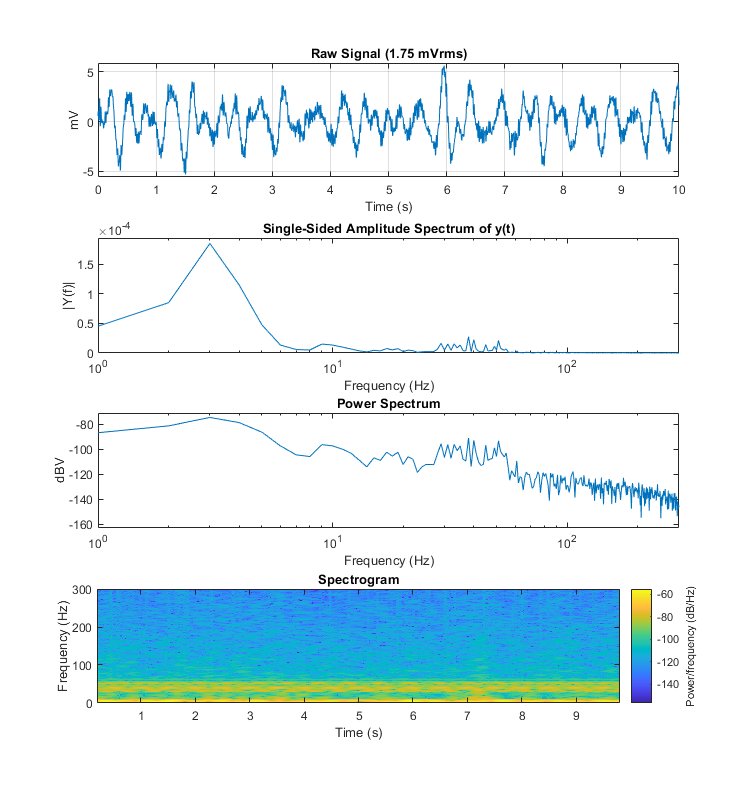# LFP Plot Example

Import streaming LFP data into MATLAB using TDTbin2mat
Plot the power spectrum and RMS of the waveform
Good for sleep scoring and behavioral discrimination

## Housekeeping

Clear workspace and close existing figures. Add SDK directories to MATLAB path.

``````close all; clear all; clc;
[MAINEXAMPLEPATH,name,ext] = fileparts(cd); % \TDTMatlabSDK\Examples
DATAPATH = fullfile(MAINEXAMPLEPATH, 'ExampleData'); % \TDTMatlabSDK\Examples\ExampleData
[SDKPATH,name,ext] = fileparts(MAINEXAMPLEPATH); % \TDTMatlabSDK
``````

## Importing the Data

This example assumes you downloaded our example data sets and extracted it into the \TDTMatlabSDK\Examples directory. To import your own data, replace 'BLOCKPATH' with the path to your own data block.

In Synapse, you can find the block path in the database. Go to Menu → History. Find your block, then Right-Click → Copy path to clipboard.

``````BLOCKPATH = fullfile(DATAPATH,'Algernon-180308-130351');
``````

Let's pick the store name, channel, and how much data we want to extract, in seconds.

``````STORE = 'LFP1';
CHANNEL = 1;
T2 = 10;
``````

Now read channel 1 from all stream data into a MATLAB structure called 'data'.

``````data = TDTbin2mat(BLOCKPATH, 'TYPE', {'streams'}, 'STORE', STORE, 'CHANNEL', CHANNEL, 'T2', T2);
``````
``````read from t=0.00s to t=10.00s
``````

And that's it! Your data is now in MATLAB. The rest of the code is a plotting example.

## LFP Analysis

Let's create a power spectrum and spectrogram plot of our data in the 0-300 Hz range.

``````TDTfft(data.streams.(STORE), 1, 'SPECPLOT', 1, 'FREQ', [0 300]);

% Make the figure larger
set(gcf,'Position',[100 100 750 800])
``````